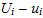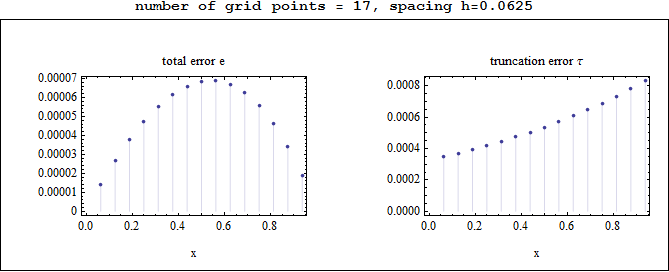Comparing total error against truncation error at each grid point resulting from the numerical solution of u''(x)=exp(x) using finite difference discretization

Nasser M. Abbasi, 10/25/2010

Introduction

The differential equationwith Dirichlet boundary conditions u=0,u=0 is solved numerically by approximating u''[x] bywhere h is the spacing between grid points. The truncation error τ at eachis given bywhile the total error e at the same point is given bywhereis the numerical solution found at that point andis the exact solution u(x) evaluated at the same point.
A small program is written to show the distribution of e and τ along the length of the domain [0,1] as h is made smaller and smaller.
This was run for few iterations, where h was divided by half each time, the system was solved for U and the result is shown in a series of plots.

ResultsObservations on the above plots

The total error e was largest in the middle of the grid, and smallest at the edges, while the truncation error was smallest at the left edge and largest at the right edge.
Comparing e to τ on each grid point is useful to understand the distribution of errors along the domain, but for verification that the numerical solution converges to the exact solution as h becomes smaller, the grid norm of e is the one examined to verify that it is less than or equal to the grid norm of τ.
Therefore, the following plots are generated which shows howcompares toas h becomes smaller. These norms are grid norms and not the standard norms.Observations on the above plots

The total error has smaller grid norm. This shows convergence, since this implies that ||e||, therefore showing that=O(1) which is the condition for convergence. The above results confirms this for this problem.